# RD Sharma Solutions for Class 8 Maths Chapter 22 Mensuration - III (Surface Area and Volume of a Right Circular Cylinder) Exercise 22.1

In Exercise 22.1 of Chapter 22, we shall discuss concepts related to a right circular cylinder, the surface area of a right circular cylinder and surface area of a hollow cylinder (a solid bounded by two coaxial cylinders of the same height and different radii is termed as a hollow cylinder). Solutions to any exercise wise problem of any chapter can be referred from RD Sharma Class 8 Solutions according to students requirements. Our experts have derived the solutions in a step by step format to help students understand the concepts clearly. Additionally, students can download the pdf from the links provided below.

## Download the Pdf of RD Sharma Solutions for Class 8 Maths Exercise 22.1 Chapter 22 Mensuration – III (Surface Area and Volume of a Right Circular Cylinder)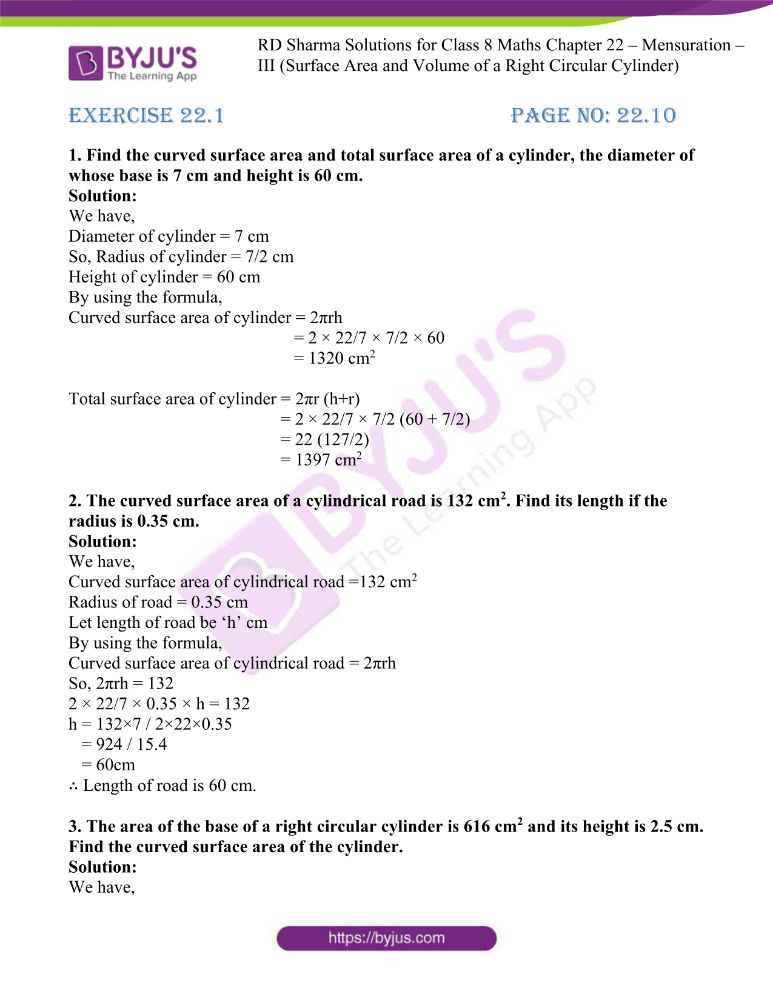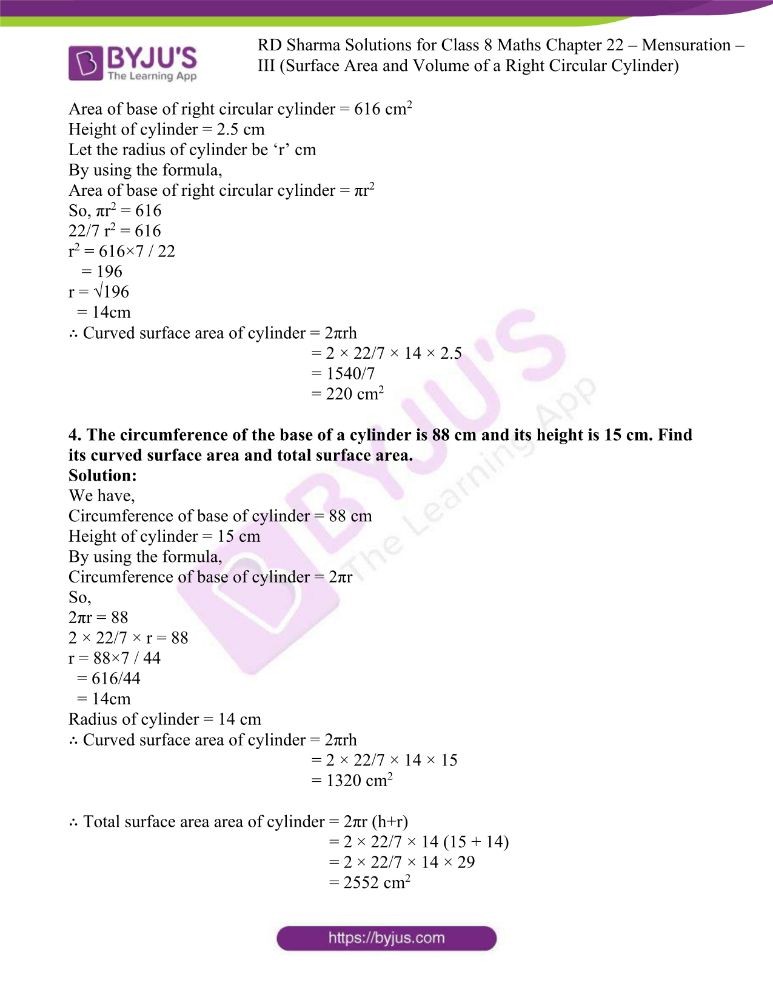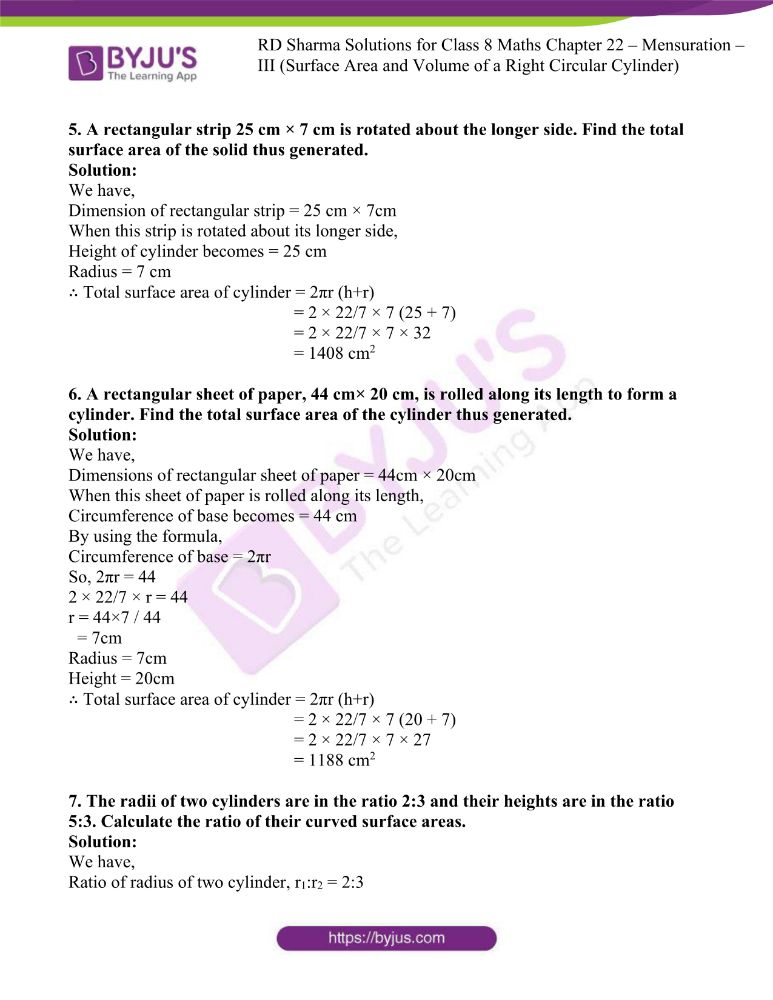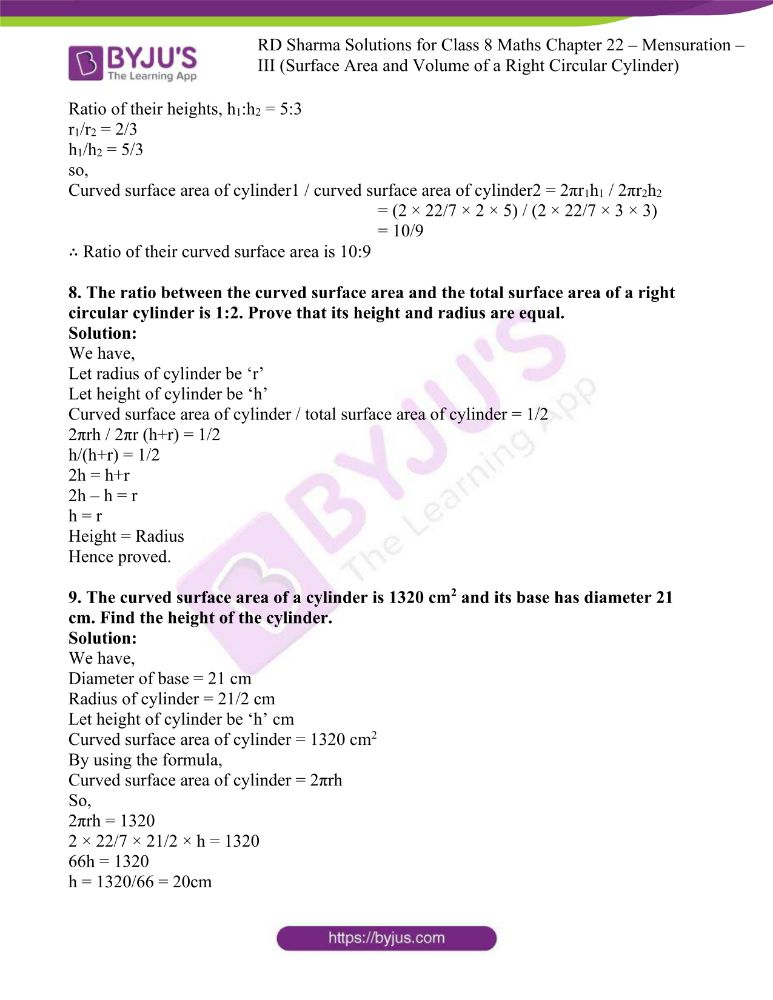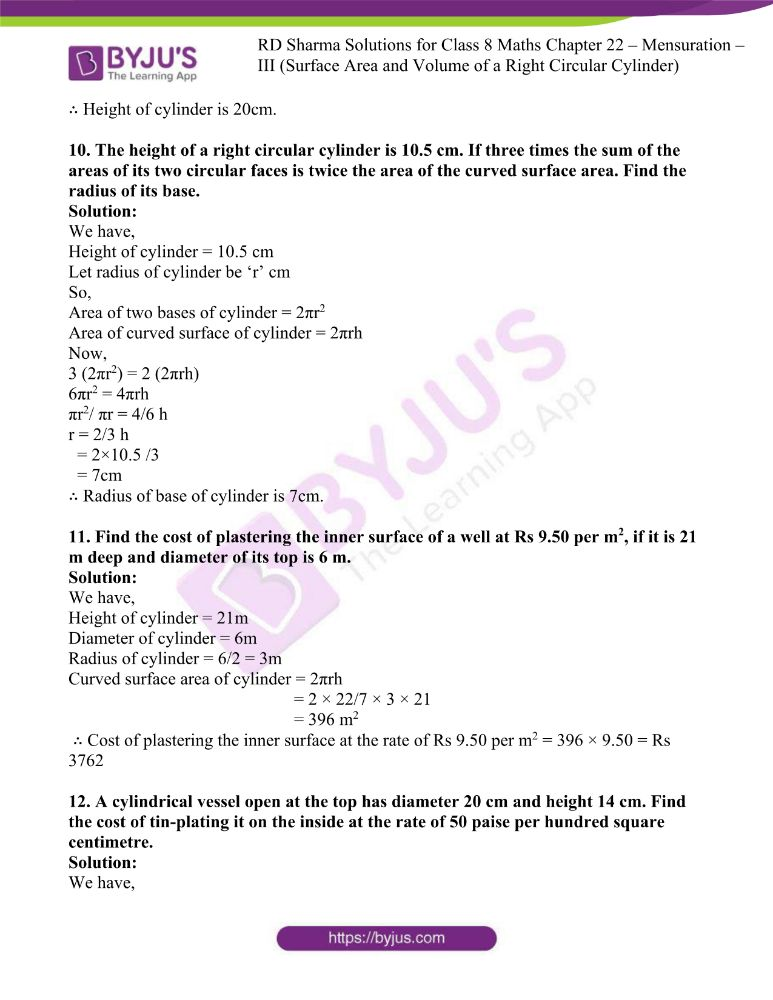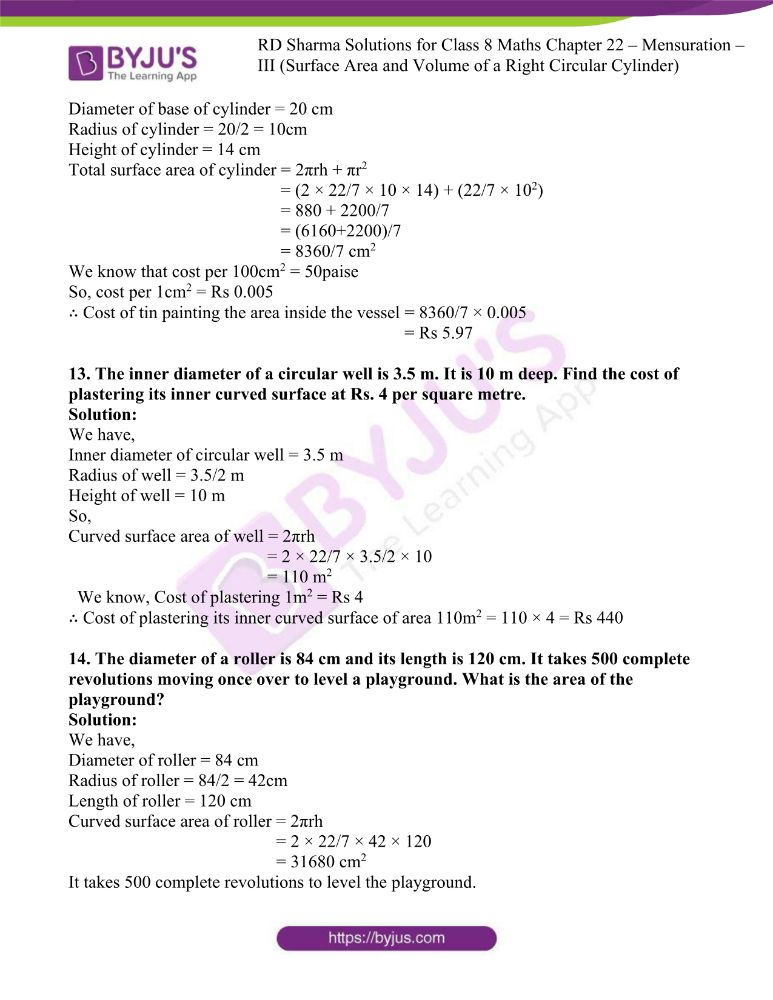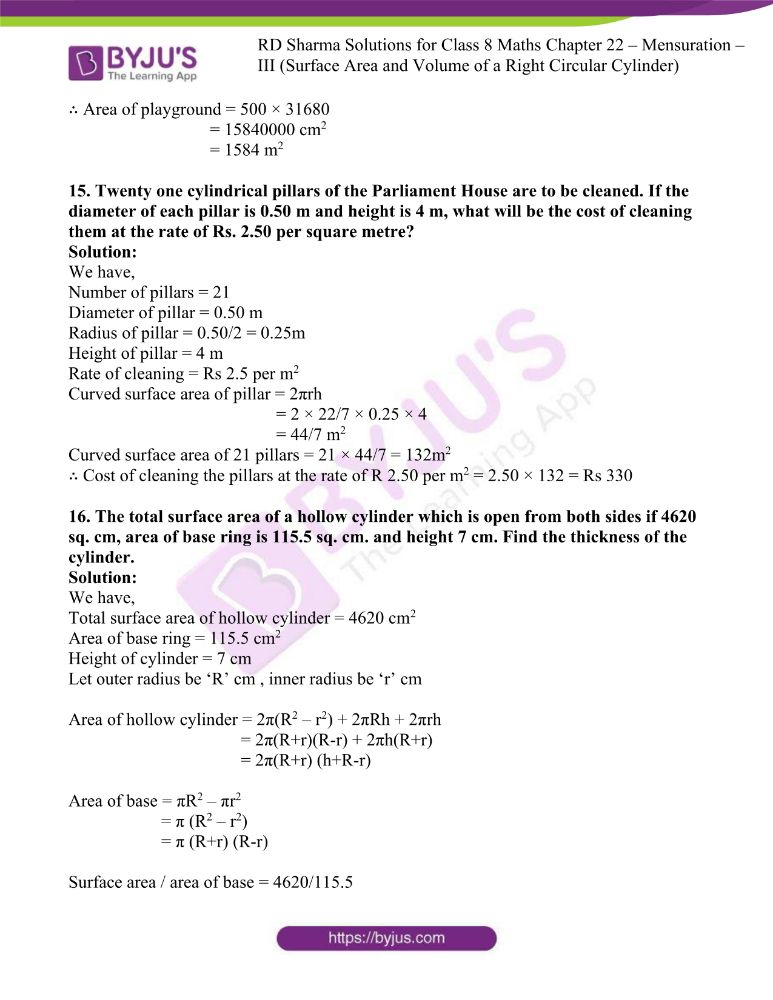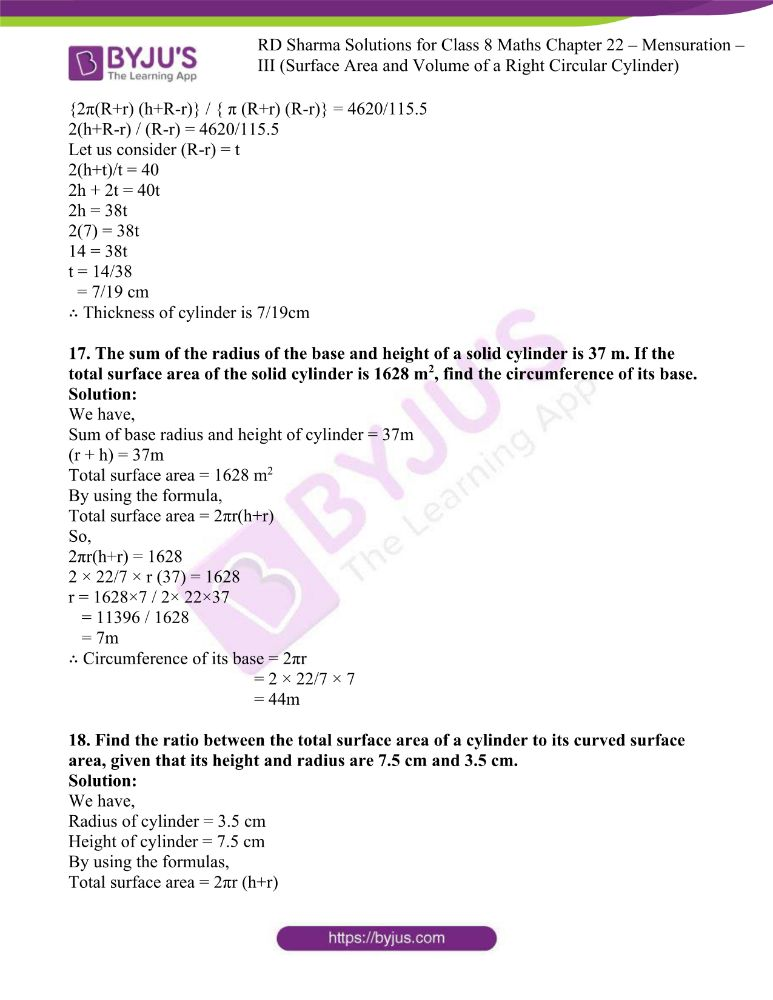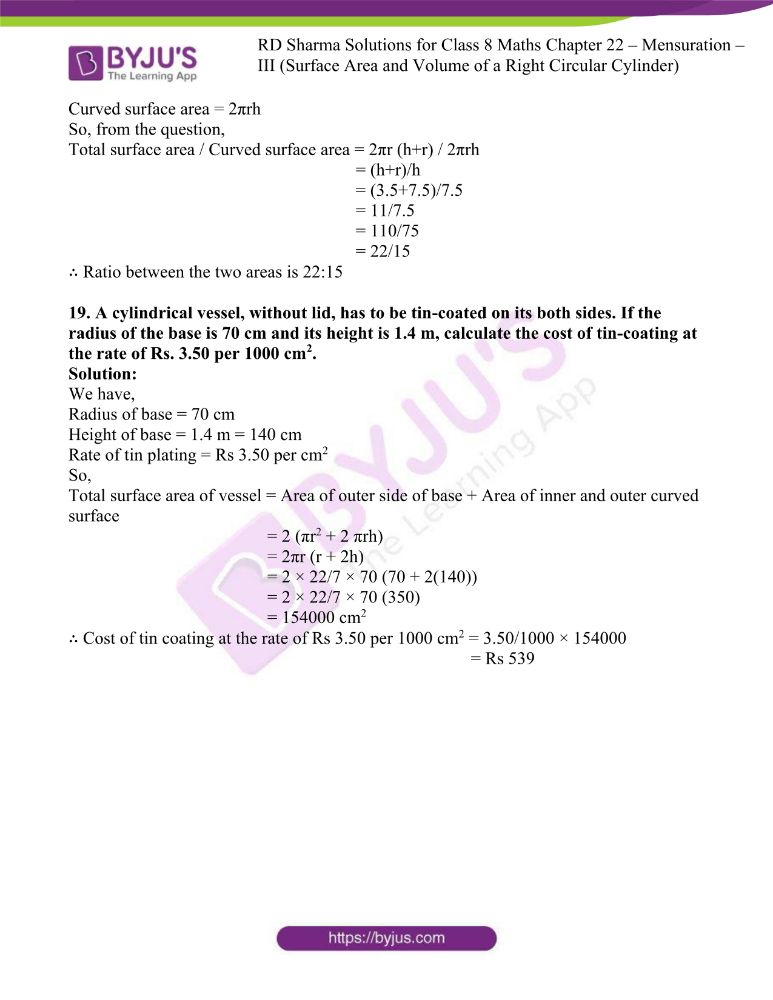### Access another exercise of RD Sharma Class 8 Maths Solutions Chapter 22 Mensuration – III (Surface Area and Volume of a Right Circular Cylinder)

Exercise 22.2 Solutions

### Access answers to RD Sharma Maths Solutions For Class 8 Exercise 22.1 Chapter 22 Mensuration – II (Volumes and Surface Areas of a Cuboid and a Cube)

1. Find the curved surface area and total surface area of a cylinder, the diameter of whose base is 7 cm and height is 60 cm.

Solution:

We have,

Diameter of cylinder = 7 cm

So, Radius of cylinder = 7/2 cm

Height of cylinder = 60 cm

By using the formula,

Curved surface area of cylinder = 2πrh

= 2 × 22/7 × 7/2 × 60

= 1320 cm2

Total surface area of cylinder = 2πr (h+r)

= 2 × 22/7 × 7/2 (60 + 7/2)

= 22 (127/2)

= 1397 cm2

2. The curved surface area of a cylindrical road is 132 cm2. Find its length if the radius is 0.35 cm.

Solution:

We have,

Curved surface area of cylindrical road =132 cm2

Let length of road be ‘h’ cm

By using the formula,

Curved surface area of cylindrical road = 2πrh

So, 2πrh = 132

2 × 22/7 × 0.35 × h = 132

h = 132×7 / 2×22×0.35

= 924 / 15.4

= 60cm

∴ Length of road is 60 cm.

3. The area of the base of a right circular cylinder is 616 cm2 and its height is 2.5 cm. Find the curved surface area of the cylinder.

Solution:

We have,

Area of base of right circular cylinder = 616 cm2

Height of cylinder = 2.5 cm

Let the radius of cylinder be ‘r’ cm

By using the formula,

Area of base of right circular cylinder = πr2

So, πr2 = 616

22/7 r2 = 616

r2 = 616×7 / 22

= 196

r = √196

= 14cm

∴ Curved surface area of cylinder = 2πrh

= 2 × 22/7 × 14 × 2.5

= 1540/7

= 220 cm2

4. The circumference of the base of a cylinder is 88 cm and its height is 15 cm. Find its curved surface area and total surface area.

Solution:

We have,

Circumference of base of cylinder = 88 cm

Height of cylinder = 15 cm

By using the formula,

Circumference of base of cylinder = 2πr

So,

2πr = 88

2 × 22/7 × r = 88

r = 88×7 / 44

= 616/44

= 14cm

Radius of cylinder = 14 cm

∴ Curved surface area of cylinder = 2πrh

= 2 × 22/7 × 14 × 15

= 1320 cm2

∴ Total surface area area of cylinder = 2πr (h+r)

= 2 × 22/7 × 14 (15 + 14)

= 2 × 22/7 × 14 × 29

= 2552 cm2

5. A rectangular strip 25 cm × 7 cm is rotated about the longer side. Find the total surface area of the solid thus generated.

Solution:

We have,

Dimension of rectangular strip = 25 cm × 7cm

When this strip is rotated about its longer side,

Height of cylinder becomes = 25 cm

Radius = 7 cm

∴ Total surface area of cylinder = 2πr (h+r)

= 2 × 22/7 × 7 (25 + 7)

= 2 × 22/7 × 7 × 32

= 1408 cm2

6. A rectangular sheet of paper, 44 cm× 20 cm, is rolled along its length to form a cylinder. Find the total surface area of the cylinder thus generated.

Solution:

We have,

Dimensions of rectangular sheet of paper = 44cm × 20cm

When this sheet of paper is rolled along its length,

Circumference of base becomes = 44 cm

By using the formula,

Circumference of base = 2πr

So, 2πr = 44

2 × 22/7 × r = 44

r = 44×7 / 44

= 7cm

Height = 20cm

∴ Total surface area of cylinder = 2πr (h+r)

= 2 × 22/7 × 7 (20 + 7)

= 2 × 22/7 × 7 × 27

= 1188 cm2

7. The radii of two cylinders are in the ratio 2:3 and their heights are in the ratio 5:3. Calculate the ratio of their curved surface areas.

Solution:

We have,

Ratio of radius of two cylinder, r1:r2 = 2:3

Ratio of their heights, h1:h2 = 5:3

r1/r2 = 2/3

h1/h2 = 5/3

so,

Curved surface area of cylinder1 / curved surface area of cylinder2 = 2πr1h1 / 2πr2h2

= (2 × 22/7 × 2 × 5) / (2 × 22/7 × 3 × 3)

= 10/9

∴ Ratio of their curved surface area is 10:9

8. The ratio between the curved surface area and the total surface area of a right circular cylinder is 1:2. Prove that its height and radius are equal.

Solution:

We have,

Let radius of cylinder be ‘r’

Let height of cylinder be ‘h’

Curved surface area of cylinder / total surface area of cylinder = 1/2

2πrh / 2πr (h+r) = 1/2

h/(h+r) = 1/2

2h = h+r

2h – h = r

h = r

Hence proved.

9. The curved surface area of a cylinder is 1320 cm2 and its base has diameter 21 cm. Find the height of the cylinder.

Solution:

We have,

Diameter of base = 21 cm

Radius of cylinder = 21/2 cm

Let height of cylinder be ‘h’ cm

Curved surface area of cylinder = 1320 cm2

By using the formula,

Curved surface area of cylinder = 2πrh

So,

2πrh = 1320

2 × 22/7 × 21/2 × h = 1320

66h = 1320

h = 1320/66 = 20cm

∴ Height of cylinder is 20cm.

10. The height of a right circular cylinder is 10.5 cm. If three times the sum of the areas of its two circular faces is twice the area of the curved surface area. Find the radius of its base.

Solution:

We have,

Height of cylinder = 10.5 cm

Let radius of cylinder be ‘r’ cm

So,

Area of two bases of cylinder = 2πr2

Area of curved surface of cylinder = 2πrh

Now,

3 (2πr2) = 2 (2πrh)

6πr2 = 4πrh

πr2/ πr = 4/6 h

r = 2/3 h

= 2×10.5 /3

= 7cm

∴ Radius of base of cylinder is 7cm.

11. Find the cost of plastering the inner surface of a well at Rs 9.50 per m2, if it is 21 m deep and diameter of its top is 6 m.

Solution:

We have,

Height of cylinder = 21m

Diameter of cylinder = 6m

Radius of cylinder = 6/2 = 3m

Curved surface area of cylinder = 2πrh

= 2 × 22/7 × 3 × 21

= 396 m2

∴ Cost of plastering the inner surface at the rate of Rs 9.50 per m2 = 396 × 9.50 = Rs 3762

12. A cylindrical vessel open at the top has diameter 20 cm and height 14 cm. Find the cost of tin-plating it on the inside at the rate of 50 paise per hundred square centimetre.

Solution:

We have,

Diameter of base of cylinder = 20 cm

Radius of cylinder = 20/2 = 10cm

Height of cylinder = 14 cm

Total surface area of cylinder = 2πrh + πr2

= (2 × 22/7 × 10 × 14) + (22/7 × 102)

= 880 + 2200/7

= (6160+2200)/7

= 8360/7 cm2

We know that cost per 100cm2 = 50paise

So, cost per 1cm2 = Rs 0.005

∴ Cost of tin painting the area inside the vessel = 8360/7 × 0.005

= Rs 5.97

13. The inner diameter of a circular well is 3.5 m. It is 10 m deep. Find the cost of plastering its inner curved surface at Rs. 4 per square metre.

Solution:

We have,

Inner diameter of circular well = 3.5 m

Radius of well = 3.5/2 m

Height of well = 10 m

So,

Curved surface area of well = 2πrh

= 2 × 22/7 × 3.5/2 × 10

= 110 m2

We know, Cost of plastering 1m2 = Rs 4

∴ Cost of plastering its inner curved surface of area 110m2 = 110 × 4 = Rs 440

14. The diameter of a roller is 84 cm and its length is 120 cm. It takes 500 complete revolutions moving once over to level a playground. What is the area of the playground?

Solution:

We have,

Diameter of roller = 84 cm

Radius of roller = 84/2 = 42cm

Length of roller = 120 cm

Curved surface area of roller = 2πrh

= 2 × 22/7 × 42 × 120

= 31680 cm2

It takes 500 complete revolutions to level the playground.

∴ Area of playground = 500 × 31680

= 15840000 cm2

= 1584 m2

15. Twenty one cylindrical pillars of the Parliament House are to be cleaned. If the diameter of each pillar is 0.50 m and height is 4 m, what will be the cost of cleaning them at the rate of Rs. 2.50 per square metre?

Solution:

We have,

Number of pillars = 21

Diameter of pillar = 0.50 m

Radius of pillar = 0.50/2 = 0.25m

Height of pillar = 4 m

Rate of cleaning = Rs 2.5 per m2

Curved surface area of pillar = 2πrh

= 2 × 22/7 × 0.25 × 4

= 44/7 m2

Curved surface area of 21 pillars = 21 × 44/7 = 132m2

∴ Cost of cleaning the pillars at the rate of R 2.50 per m2 = 2.50 × 132 = Rs 330

16. The total surface area of a hollow cylinder which is open from both sides if 4620 sq. cm, area of base ring is 115.5 sq. cm. and height 7 cm. Find the thickness of the cylinder.

Solution:

We have,

Total surface area of hollow cylinder = 4620 cm2

Area of base ring = 115.5 cm2

Height of cylinder = 7 cm

Let outer radius be ‘R’ cm , inner radius be ‘r’ cm

Area of hollow cylinder = 2π(R2 – r2) + 2πRh + 2πrh

= 2π(R+r)(R-r) + 2πh(R+r)

= 2π(R+r) (h+R-r)

Area of base = πR2 – πr2

= π (R2 – r2)

= π (R+r) (R-r)

Surface area / area of base = 4620/115.5

{2π(R+r) (h+R-r)} / { π (R+r) (R-r)} = 4620/115.5

2(h+R-r) / (R-r) = 4620/115.5

Let us consider (R-r) = t

2(h+t)/t = 40

2h + 2t = 40t

2h = 38t

2(7) = 38t

14 = 38t

t = 14/38

= 7/19 cm

∴ Thickness of cylinder is 7/19cm

17. The sum of the radius of the base and height of a solid cylinder is 37 m. If the total surface area of the solid cylinder is 1628 m2, find the circumference of its base.

Solution:

We have,

Sum of base radius and height of cylinder = 37m

(r + h) = 37m

Total surface area = 1628 m2

By using the formula,

Total surface area = 2πr(h+r)

So,

2πr(h+r) = 1628

2 × 22/7 × r (37) = 1628

r = 1628×7 / 2× 22×37

= 11396 / 1628

= 7m

∴ Circumference of its base = 2πr

= 2 × 22/7 × 7

= 44m

18. Find the ratio between the total surface area of a cylinder to its curved surface area, given that its height and radius are 7.5 cm and 3.5 cm.

Solution:

We have,

Radius of cylinder = 3.5 cm

Height of cylinder = 7.5 cm

By using the formulas,

Total surface area = 2πr (h+r)

Curved surface area = 2πrh

So, from the question,

Total surface area / Curved surface area = 2πr (h+r) / 2πrh

= (h+r)/h

= (3.5+7.5)/7.5

= 11/7.5

= 110/75

= 22/15

∴ Ratio between the two areas is 22:15

19. A cylindrical vessel, without lid, has to be tin-coated on its both sides. If the radius of the base is 70 cm and its height is 1.4 m, calculate the cost of tin-coating at the rate of Rs. 3.50 per 1000 cm2.

Solution:

We have,

Radius of base = 70 cm

Height of base = 1.4 m = 140 cm

Rate of tin plating = Rs 3.50 per cm2

So,

Total surface area of vessel = Area of outer side of base + Area of inner and outer curved surface

= 2 (πr2 + 2 πrh)

= 2πr (r + 2h)

= 2 × 22/7 × 70 (70 + 2(140))

= 2 × 22/7 × 70 (350)

= 154000 cm2

∴ Cost of tin coating at the rate of Rs 3.50 per 1000 cm2 = 3.50/1000 × 154000

= Rs 539